Short Answers Type Questions- Heron’s Formula

# Short Answers Type Questions- Heron’s Formula - Mathematics (Maths) Class 9

Q1. The area of an equilateral triangle whose side is ‘a’ cm is (√3/4)acm2. Find its height.

Area of the triangle = (√3/4)a2 cm2
∵ Area of a triangle = (1/2)x base x height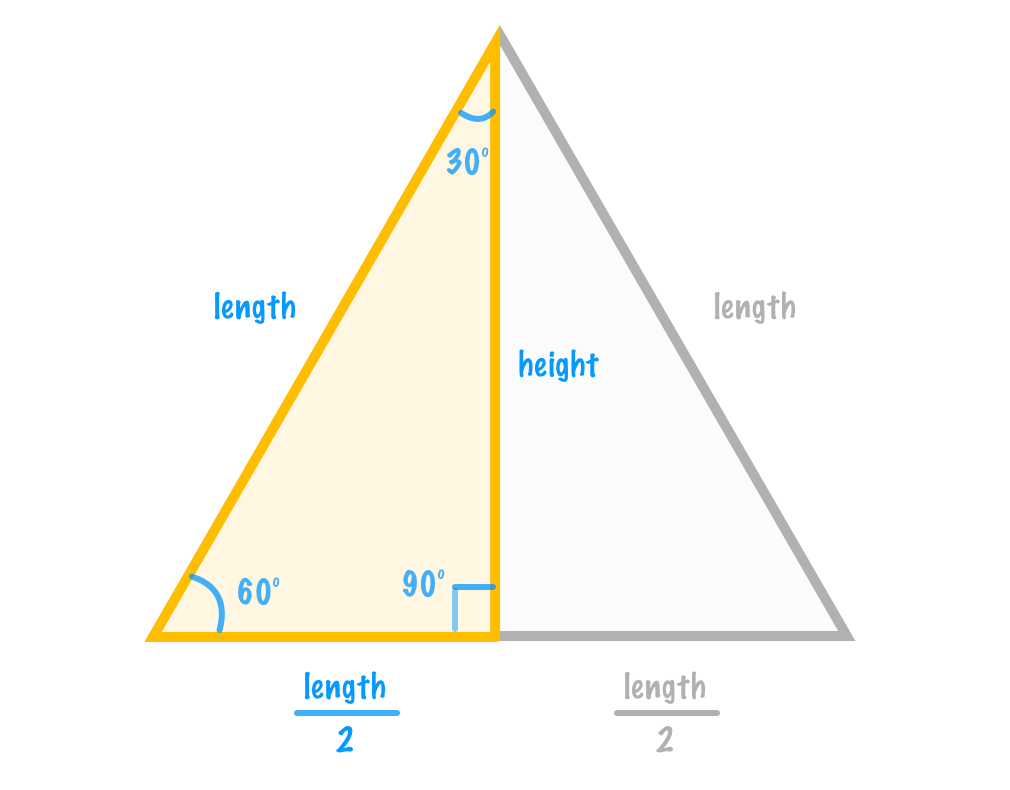∴  (1/2) x a x height =(√3/4)x a2
⇒ height =(√3/4) a2 x (2/a)  = (√3/2) a cm

Q2. Find the height of an equilateral triangle whose side is 2 cm.

Since height of an equilateral triangle is given by
height = (√3/2) x side
⇒ height =(√3/2) x 2 cm = 3 cm

Q3. Find the length of a diagonal of a square whose side is 2 cm.

The diagonal of a square = (√2) a cm
∴ Length of the diagonal = (√2) x 2 cm = 2√2cm.

Q4. The diagonal of a square is 9√2 cm. What is the side?

Let side of the square = x cm.
∵ Its diagonal is given by √2 x side.
∴ √2 x x= 9 x √2

⇒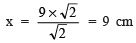Thus, the required length of sides of the square is 9 cm.

Q5. The length of a rectangular plot of land is twice its breadth. If the perimeter of the plot be 180 metres, then find its area.

Let the breadth of the plot be ‘x’ metres.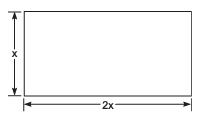∴ Its length = 2x metres
Since perimeter of a rectangular plot = 2[Length + Breadth]
∴ Perimeter of the given plot = 2[x + 2x]
= 2[3x]
= 6x metres
⇒ 6x = 180
⇒ x=  180/6= 30 metres
⇒ 2x = 2 x 30 = 60 metres.
∴ Length of the plot = 60 metres and Breadth of the plot = 30 meters.
∴ Area of the plot = Length x Breadth = 60 x 30 m2 = 1800 m

Q6. The length of the sides containing the right angle in a  right triangle differ by 7 cm. The area of the triangle is 60 cm2. Find the length of the hypotenuse.

Let the sides containing the right angle be ‘x’ cm and (x – 7) cm.
i.e. Base = x cm and height = (x – 7) cm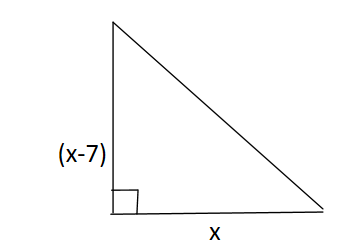∴ Area = (1/2) x base x height=(1/2) x x x (x – 7) cm2
Now (1/2) x (x – 7) = 60
⇒ x(x – 7) = 120
⇒ x2 – 7x – 120 = 0
⇒ x2 – 15x + 8x – 120 = 0
⇒ x(x – 15) + 8(x – 15) = 0
⇒ (x + 8)(x – 15) = 0
⇒ x = – 8 or x = 15
Rejecting x = –8, we have x – 15 = 0
⇒ x = 15 cm x – 7 = 15 – 7 = 8 cm
Now, Hypotenuse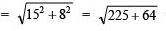= √289 = 17cm
Thus, the required length of the hypotenuse is 17 cm.

Q7. The lengths of sides of a triangle are in the ratio 3 : 4 : 5 and its perimeter is 120 cm, find its area.

The sides are in the ratio of 3 : 4 : 5.
Let the sides be 3x, 4x and 5x.
∴ Perimeter = 3x + 4x + 5x = 12x
Now 12x = 120                [Perimeter = 120 cm]
⇒   x =(120/12) = 10
∴ Lengths are: a = 3x = 3 x 10 = 30 cm
b = 4x = 4 x 10 = 40 cm
c = 5x = 5 x 10 = 50 cm
Now, semi-perimeter (s) = (120/12)
cm = 60 cm
∵ (s – a) = 60 – 30 = 30 cm
(s – b) = 60 – 40 = 20 cm
(s – c) = 60 – 50 = 10 cm

Using Heron’s formula, we have

Area of the triangle =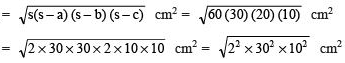= 2 x 30 x 10 cm2 = 600 cm2 Thus, the required area of the triangle = 600 cm2.

Q8. Find the area of a quadrilateral ABCD in which AB = 8 cm, BC = 6 cm, CD = 8 cm, DA = 10 cm and AC = 10 cm.

In ΔABC, ∠B = 90°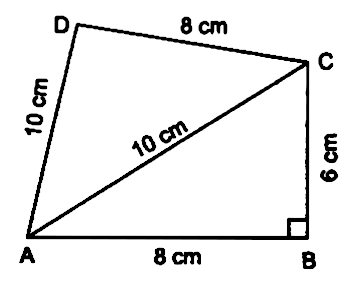∴ area of right (rt ΔABC) = (1/2) x 8 x 6 cm2 = 24 cm2
In ΔACD,
a = AC = 10 cm b = AD = 10 cm c = CD = 8 cm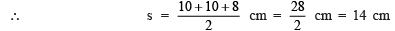∴Area of ΔACD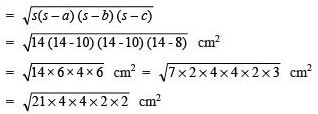= 2 x 4√21 = 8√21 cm2

= 8 x 4,58 cm2 = 36.64 cm2

Now, area of quadrilateral ABCD = ar (ΔABC) + ar (ΔACD)
= 24 cm2 + 8√21 cm
= 24 cm2 + 36.64 cm2
= 60.64 cm2

Q9. How much paper of each shade is needed to make a kite given in the figure, in which ABCD is a square of diagonal 44 cm.

∵ The diagonals of a square bisect each other at right angles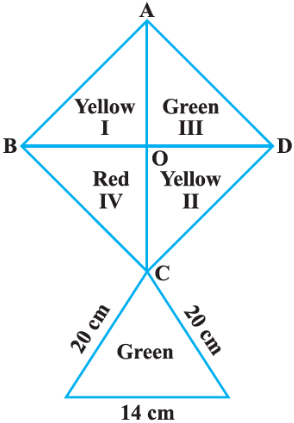∴ OB = OD = OA = OC = (44/2) = 22 cm
Now, ar rt (Δ –I) = (1/2)× OB × OA
= (1/2) × 22 × 22 cm2 = 242 cm2
Similarly ar rt (Δ –II) = arrt(Δ–III) = ar rt (Δ –IV) = 242 cm2

∵ Sides of ΔCEF are 20 cm, 20 cm and 14 cm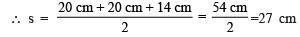⇒ Area of ΔCEF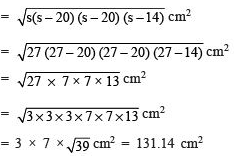Now, area of yellow paper = ar (Δ – I) + ar (Δ – II)
= 242 cm+ 242 cm2 = 484 cm2
Area of red paper = ar (Δ – IV) = 242 cm2
Area of green paper = ar (Δ – III) + ar ΔCEF
= 242 cm2 + 131.14 cm2
= 373.14 cm2

The document Short Answers Type Questions- Heron’s Formula | Mathematics (Maths) Class 9 is a part of the Class 9 Course Mathematics (Maths) Class 9.
All you need of Class 9 at this link: Class 9

## FAQs on Short Answers Type Questions- Heron’s Formula - Mathematics (Maths) Class 9

 1. What is Heron's formula and how is it used to find the area of a triangle?Ans. Heron's formula is used to find the area of a triangle when the lengths of all three sides are known. The formula is as follows: Area = √(s(s-a)(s-b)(s-c)), where s is the semi-perimeter of the triangle and a, b, and c are the lengths of the sides. To find the area using Heron's formula, first calculate the semi-perimeter by adding all three side lengths and dividing by 2. Then substitute the values into the formula and simplify to find the area.
 2. How is Heron's formula derived?Ans. Heron's formula is derived using a combination of algebraic manipulations and the concept of area. It can be derived by dividing the triangle into two right triangles and then using the formula for the area of a triangle (1/2 * base * height) for each right triangle. By substituting the base and height expressions in terms of the side lengths, simplifying the equation, and applying some algebraic manipulations, Heron's formula can be obtained.
 3. Can Heron's formula be used for all types of triangles?Ans. Yes, Heron's formula can be used for all types of triangles, including scalene, isosceles, and equilateral triangles. It can be applied when the lengths of all three sides are known.
 4. Are there any limitations or conditions for using Heron's formula?Ans. Yes, there are a few conditions for using Heron's formula. First, the lengths of all three sides must be positive real numbers. Second, the triangle must be a valid triangle, meaning that the sum of any two sides must be greater than the third side. If these conditions are not met, Heron's formula cannot be used to find the area.
 5. Can Heron's formula be used to find the area of a triangle given only the lengths of two sides and an angle?Ans. No, Heron's formula cannot be used to find the area of a triangle given only the lengths of two sides and an angle. Heron's formula requires the lengths of all three sides to calculate the area accurately. If only the lengths of two sides and an angle are known, other methods such as the sine or cosine rule would need to be applied to find the area of the triangle.

## Mathematics (Maths) Class 9

62 videos|426 docs|102 tests

## Mathematics (Maths) Class 9

62 videos|426 docs|102 tests
Signup to see your scores go up within 7 days! Learn & Practice with 1000+ FREE Notes, Videos & Tests.
10M+ students study on EduRev
Track your progress, build streaks, highlight & save important lessons and more!(Scan QR code)
Related Searches

,

,

,

,

,

,

,

,

,

,

,

,

,

,

,

,

,

,

,

,

,

;